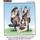## General Question# What was the last math symbol made?

Asked by RedDeerGuy1 (17704) March 14th, 2017

Who regulates the addition of new symbols?

Observing members: 0Composing members: 0I have this link if it helps.

RedDeerGuy1 (17704)“Great Answer” (0) Flag as…Nobody regulates the addition of symbols. New symbols are used to express new ideas. Knowing the symbols alone does not teach you much about math, any more than knowing the alphabet tells you much about English.

The most recent symbol that I know of is Donald Knuth’s up-arrow notation. Link allows you to represent unimaginably large numbers. I am going to sometimes use & in place of ^ because of display problems.The basic idea is fairly simple. In the beginning there was addition, like 5 + 3. This was extended to add multiple terms, like 5 + 3 4 2. When the terms being added were all the same, like 7 + 7 + 7 + 7, multiplication was devised to express this more compactly as 4*7. Multiplications, like additions could have multiple terms, and a new notation, exponentiation, was used to express this when the terms were identical.. 7 * 7 * 7 * 7 = 7&4.

Why stop there? Well there actually is a good reason, that being the lack of any real need, but when was the last time a theoretical mathematician cared about practical matters. We can have multiple exponentiations. 7&7&7&7. Knuth’s notation for this is 7^^4. It should be apparent that we can extend this process indefinitely,. 7^^7^^7^^7 = 7^^^4. One thing that should be mentioned is that the operations are carried out from right to left. For example, 7^^3 = 7&(7&7)

To get a rough idea how big these numbers get, a googol is a 1 followed by 100 zeros, or 10^100 or 10&10&2. A googol is larger than the number of subatomic particles in the visible universe. A googolplex is a 1 followed by a googol zeros or ten to the googol, or 10&10&10&2. If we replace the 2 by a 10, we get 10^^4 in Knuth’s notation. We have created a number way beyond the imagination without hardly breaking a sweat.

LostInParadise (27910)“Great Answer” (1) Flag as…You might be interested in the Metamath site, which builds mathematics from the ground up. I have only started looking at it, so I can’t tell how useful it is, but I like the idea behind it. Metamath is a computer language that can verify (but not generate) proofs. As I understand it, axioms and theorems are treated as subroutines that accept input and generate a line of characters. The subroutines can be chained together to form new new subroutines corresponding to new theorems.

LostInParadise (27910)“Great Answer” (1) Flag as…or